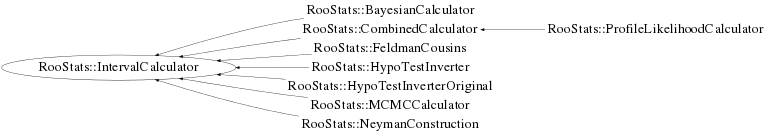# class RooStats::IntervalCalculator

```
```

IntervalCalculator is an interface class for a tools which produce RooStats ConfIntervals. The interface currently assumes that any interval calculator can be configured by specifying:

• a model,
• a data set,
• a set of parameters of interest,
• a set of nuisance parameters (eg. parameters on which the model depends, but are not of interest), and
• a confidence level or size of the test (eg. rate of Type I error).
The interface allows one to pass the model, data, and parameters via a workspace and then specify them with names. The interface will be extended so that one does not need to use a workspace.

After configuring the calculator, one only needs to ask GetInterval, which will return a ConfInterval pointer.

The concrete implementations of this interface should deal with the details of how the nuisance parameters are dealt with (eg. integration vs. profiling) and which test-statistic is used (perhaps this should be added to the interface).

The motivation for this interface is that we hope to be able to specify the problem in a common way for several concrete calculators.

```

```

## Function Members (Methods)

This is an abstract class, constructors will not be documented.
Look at the header to check for available constructors.

public:
 virtual ~IntervalCalculator() static TClass* Class() virtual Double_t ConfidenceLevel() const virtual RooStats::ConfInterval* GetInterval() const RooStats::IntervalCalculator IntervalCalculator() RooStats::IntervalCalculator IntervalCalculator(const RooStats::IntervalCalculator&) virtual TClass* IsA() const RooStats::IntervalCalculator& operator=(const RooStats::IntervalCalculator&) virtual void SetConfidenceLevel(Double_t cl) virtual void SetData(RooAbsData&) virtual void SetModel(const RooStats::ModelConfig&) virtual void SetTestSize(Double_t size) virtual void ShowMembers(TMemberInspector& insp) const virtual Double_t Size() const virtual void Streamer(TBuffer&) void StreamerNVirtual(TBuffer& ClassDef_StreamerNVirtual_b)

## Class Charts## Function documentation

virtual ~IntervalCalculator()
`{}`
ConfInterval* GetInterval() const
``` Main interface to get a ConfInterval, pure virtual
```
Double_t Size() const
``` Get the size of the test (eg. rate of Type I error)
```
Double_t ConfidenceLevel() const
``` Get the Confidence level for the test
```
void SetData(RooAbsData& )
``` Set the DataSet ( add to the the workspace if not already there ?)
```
void SetModel(const RooStats::ModelConfig& )
``` Set the Model
```
void SetTestSize(Double_t size)
``` set the size of the test (rate of Type I error) ( e.g. 0.05 for a 95% Confidence Interval)
```
void SetConfidenceLevel(Double_t cl)
``` set the confidence level for the interval (e.g. 0.95 for a 95% Confidence Interval)
```